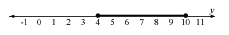### Home > CCA2 > Chapter Ch11 > Lesson 11.2.3 > Problem11-70

11-70.

Solve and graph each inequality.

1. $| 7 - y | \leq 3$

Solve this inequality like a normal equation, just use the less than symbol as the equals sign.

Remember to solve both $7 - y ≤ 3$ and $7 - y ≥ -3$.

$4 ≤ y ≤ 10$2. $3 | 2 m + 1 | - 1 > 8$

Use the same method as part (a).